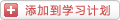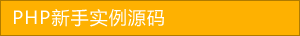﻿ 一起看看php实现产品加入购物车功能（1） - 综合实例 - PHP粉丝网

# 一起看看php实现产品加入购物车功能（1）

##### 发布:smiling　来源: PHP粉丝网 　添加日期：2022-07-12 08:34:36　浏览: 评论：0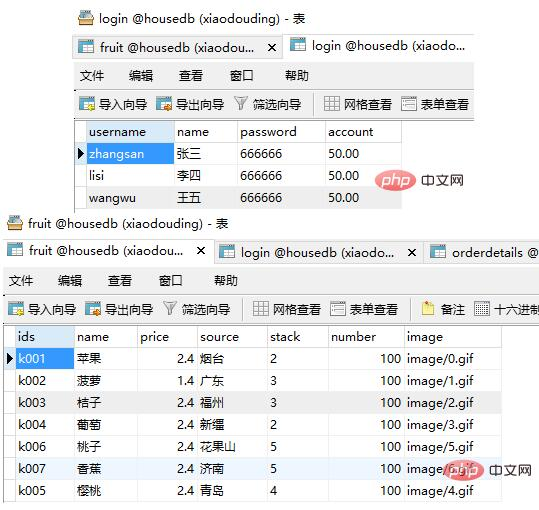1. <body>
2.
3. <form action="chuli.php" method="post"
4.
5.  <p style="margin-left: 500px; margin-top: 200px;
6.
7.   height: 250px; width: 250px; border: 1px dashed black">
8.
9.   <p style="margin-left: 100px; "><h3>登录</h3></p>
10.
11.   <p style="margin-top: 20px">用户名：<input type="text" name="uid"/></p><br/>
12.
13.   <p>密&nbsp;码：<input type="password" name="pwd"/></p><br/>
14.
15.   <p style="margin-left: 180px"><input type="submit" value="登录"/></p>
16.
17.  </p>
18.
19.
20.
21. </form>
22.
23. </body>

1. <?php
2.
3. session_start(); //开启session 必须要写到第一行
4.
6.
7.
8.
9. \$uid=\$_POST["uid"]; //从登录页面获取到用户名和密码
10.
11. \$pwd=\$_POST["pwd"];
12.
13.
14.
16.
18.
19.
20.
22.
23. \$arr=\$db->Query(\$sql);
24.
25.
26.
27. if(\$arr==\$pwd && !emptyempty(\$pwd)) //判断所填写的密码和取到的密码是一样的，而且密码不能为空
28.
29.
30.  \$_SESSION["uid"]=\$uid
31.
33.
34.
35. else
36.
37.
38.  echo"登录失败"
39.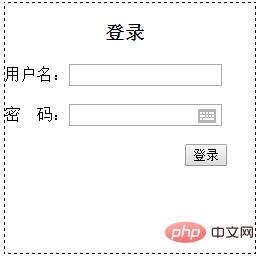1. <h2>大苹果购物网</h2>
2.
3. <?php
4.
5. session_start();
6.
8.
10.
11. ?>
12.
13. <table border="1" width="100%" cellpadding="0" cellspacing="0"
14.
15.  <tr>
16.
17.   <td>代号</td>
18.
19.   <td>水果名称</td>
20.
21.   <td>水果价格</td>
22.
23.   <td>原产地</td>
24.
25.   <td>货架</td>
26.
27.   <td>库存量</td>
28.
29.   <td></td>
30.
31.  </tr>
32.
33.  <?php
34.
35.
36.
37.  \$uid=\$_SESSION["uid"];
38.
39.
40.
41.  \$sql="select * from fruit"
42.
43.  \$arr=\$db->Query(\$sql);
44.
45.  foreach(\$arr as \$v
46.
47.  {
48.
49.   echo"<tr>
50.
51.   <td>{\$v}</td> // 从数据库调出我们所需要的内容
52.
53.   <td>{\$v}</td>
54.
55.   <td>{\$v}</td>
56.
57.   <td>{\$v}</td>
58.
59.   <td>{\$v}</td>
60.
61.   <td>{\$v}</td>
62.
63.   <td><a href='add.php?ids={\$v}'>购买</a></td> //这里的购买相当于添加购物车的功能
64.
65.  </tr>";
66.
67.  }
68.
69.  ?>
70.
71.
72.
73.  <?php
74.
75.  //这里显示的是 购物车有多少产品，和产品的总价格
76.
77.  \$ann=array();
78.
79.  if(!emptyempty(\$_SESSION["gwc"]))
80.
81.  {
82.
83.   \$ann=\$_SESSION["gwc"];
84.
85.  }
86.
87.  \$zhonglei = count(\$ann);
88.
89.
90.
91.  \$sum=0;
92.
93.  foreach(\$ann as \$k
94.
95.  {
96.
97.   \$sql1="select price from fruit where ids='{\$v}'"
98.
99.
100.
101.   \$danjia=\$db->Query(\$sql1);
102.
103.
104.
105.   foreach(\$danjia as \$n
106.
107.   {
108.
109.    \$sum=\$sum + \$n*\$k;
110.
111.   }
112.
113.
114.
115.
116.
117.  }
118.
119.  echo"购物车有<mark>{\$zhonglei}</mark>种商品，总价格为<mark>{\$sum}</mark>元"
120.
121.  ?>
122.
123. </table>
124.
125. <p>
126.
127. <a href="gouwuche.php" rel="external nofollow" rel="external nofollow" >查看购物车</a>
128.
129. <a href="main.php" rel="external nofollow" rel="external nofollow" >浏览商品</a>
130.
131. <a href="zhanghu.php" rel="external nofollow" rel="external nofollow" >查看账户</a> </p>
132.
133.
134.
135. </body>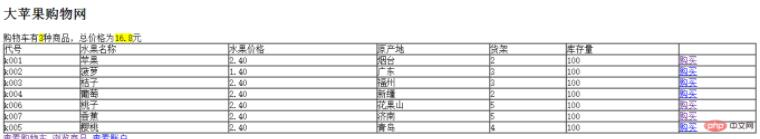1. <?php
2.
3. session_start();
4.
5.
6.
7. \$ids = \$_GET["ids"];
8.
9.
10.
11.
12.
13. if(emptyempty(\$_SESSION["gwc"]))
14.
15.
16.  //1.购物车是空的，第一次点击添加购物车
17.
18.  \$arr = array
19.
20.   array(\$ids,1)
21.
22.  );
23.
24.  \$_SESSION["gwc"]=\$arr
25.
26.
27. else
28.
29.
30.  //不是第一次点击
31.
32.  //判断购物车中是否存在该商品
33.
34.  \$arr = \$_SESSION["gwc"]; //先存一下
35.
36.
37.
38.  \$chuxian = false;
39.
40.  foreach(\$arr as \$v
41.
42.  {
43.
44.   if(\$v==\$ids
45.
46.   {
47.
48.    \$chuxian = true;
49.
50.   }
51.
52.  }
53.
54.
55.
56.  if(\$chuxian
57.
58.  {
59.
60.   //3.如果购物车中有该商品
61.
62.
63.
64.   for(\$i=0;\$i<count(\$arr);\$i++)
65.
66.   {
67.
68.    if(\$arr[\$i]==\$ids
69.
70.    {
71.
72.     \$arr[\$i]+=1;
73.
74.    }
75.
76.   }
77.
78.
79.
80.   \$_SESSION["gwc"] = \$arr
81.
82.  }
83.
84.  else
85.
86.  {
87.
88.   //2.如果购物车中没有该商品
89.
90.   \$asg = array(\$ids,1);
91.
92.   \$arr[] = \$asg
93.
94.   \$_SESSION["gwc"] = \$arr
95.
96.  }
97.
98.
99.
100.

1. <h2>购物车中有以下商品：</h2>
2.
3. <table cellpadding="0" cellspacing="0" border="1" width="100%"
4.
5.  <tr>
6.
7.   <td>商品名称</td>
8.
9.   <td>商品单价</td>
10.
11.   <td>购买数量</td>
12.
13.   <td></td>
14.
15.  </tr>
16.
17.  <?php
18.
19.  session_start();
20.
21.  //\$uid=\$_SESSION["uid"];
22.
23.
24.
25.  \$arr=array();
26.
27.
28.
29.  if(!emptyempty(\$_SESSION["gwc"]))
30.
31.  {
32.
33.   \$arr=\$_SESSION["gwc"];
34.
35.  }
36.
38.
40.
41.
42.
43.  foreach(\$arr as \$v
44.
45.  {
46.
47.   global \$db
48.
49.   \$sql="select * from fruit where ids='{\$v}'"
50.
51.   \$att=\$db -> Query(\$sql,1);
52.
53.   foreach(\$att as \$n
54.
55.   {
56.
57.    echo"<tr>
58.
59.   <td>{\$n}</td>
60.
61.   <td>{\$n}</td>
62.
63.   <td>{\$v}</td>
64.
65.   <td><a href='shanchu.php?ids={\$v}'>删除</a></td>
66.
67.  </tr>";}
68.
69.
70.
71.  }
72.
73.  ?>
74.
75.
76.
77. </table>
78.
79. <p>
80.
81. <a href="gouwuche.php" rel="external nofollow" rel="external nofollow" >查看购物车</a>
82.
83. <a href="main.php" rel="external nofollow" rel="external nofollow" >浏览商品</a>
84.
85. <a href="zhanghu.php" rel="external nofollow" rel="external nofollow" >查看账户</a> </p> 14 15 </body>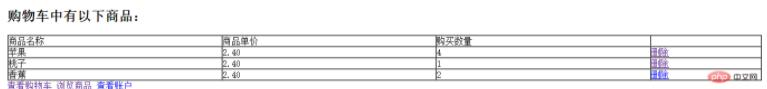### 分享到： document.write('<a href="http://v.t.sina.com.cn/share/share.php?url='+encodeURIComponent(location.href)+'&appkey=3172366919&title='+encodeURIComponent('一起看看php实现产品加入购物车功能（1）')+'" title="分享到新浪微博" class="t1" target="_blank">&nbsp;</a>'); document.write('<a href="http://www.douban.com/recommend/?url='+encodeURIComponent(location.href)+'&title='+encodeURIComponent('一起看看php实现产品加入购物车功能（1）')+'" title="分享到豆瓣" class="t2" target="_blank">&nbsp;</a>'); document.write('<a href="http://share.renren.com/share/buttonshare.do?link='+encodeURIComponent(location.href)+'&title='+encodeURIComponent('一起看看php实现产品加入购物车功能（1）')+'" title="分享到人人" class="t3" target="_blank">&nbsp;</a>'); document.write('<a href="http://www.kaixin001.com/repaste/share.php?rtitle='+encodeURIComponent('一起看看php实现产品加入购物车功能（1）')+'&rurl='+encodeURIComponent(location.href)+'&rcontent=" title="分享到开心网" class="t4" target="_blank">&nbsp;</a>'); document.write('<a href="http://sns.qzone.qq.com/cgi-bin/qzshare/cgi_qzshare_onekey?url='+encodeURIComponent(location.href)+'" title="分享到QQ空间" class="t5" target="_blank">&nbsp;</a>');